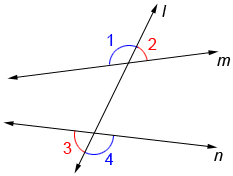# Alternate Exterior Definition

. . . . .

If a straight line crosses the two parallel lines, then this intersection of lines is called a transversal in mathematics. When coplanar lines or parallel lines cut the transversal, some angles at the outer edges are formed, known as alternate exterior angles.

These Alternate angles are the non-adjacent combination of angles formed at each side of the transversal.

When two parallel lines crossed by transversal the pair of an angle formed between the lines are equivalent to each other.

For more precise understanding let's see the diagram listed below:Note:

If you want to know about alternate interior angle, you can use our Alternate Interior Angle Definition.

Here you can see the alternate exterior angles formed between two parallel lines are equal to each other.